# Logarithmic oscillator thermostat

The Logarithmic oscillator  in one dimension is given by (Eq. 2):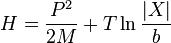$H = \frac{P^2}{2M}+ T \ln \frac{\vert X \vert}{b}$

where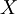$X$ is the position of the logarithmic oscillator,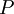$P$ is its linear momentum, and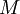$M$ represents its mass.$T$ is the desired temperature of the thermostat, and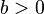$b > 0$ sets a length-scale.

## As a thermostat

From the Virial theorem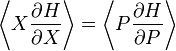$\left\langle X\frac{\partial H}{\partial X} \right\rangle = \left\langle P\frac{\partial H}{\partial P} \right\rangle$

one obtains$T = \left\langle \frac{P^2}{M} \right\rangle$.

This implies that all expectation values of the trajectories correspond to the very same temperature of the thermostat, irrespective of the internal energy. In other words,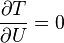$\frac{\partial T}{\partial U} = 0$

this implies that the heat capacity becomes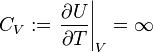$C_V := \left. \frac{\partial U}{\partial T} \right\vert_V = \infty$

Having an infinite heat capacity is an ideal feature for a thermostat.

## Practical applicability

The averages considered above make sense only for times greater than the period of the logarithmic oscillator but, because of the logarithmic shape of the potential, the period increases proportionally to the exponential of the total energy . That is to say, if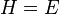$H = E$, then the period of oscillation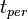$t_{per}$ increases with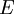$E$ according to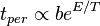$t_{per} \propto b e^{E/T}$. Furthermore, the maximum excursions of the oscillator also move outwards exponentially,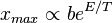$x_{max} \propto be^{E/T}$. This exponential scaling of time and length scales severely limits the practical applicability of the logarithmic thermostat . Campisi et al. have defended that such a thermostat could work when interacting weakly with small atomic clusters , but further research has shown that the logarithmic oscillator does not generally behave as a thermostat even in that setting . In addition, when two logarithmic oscillators with different values of$T$ interact weakly with a system, they fail to promote heat flow  .